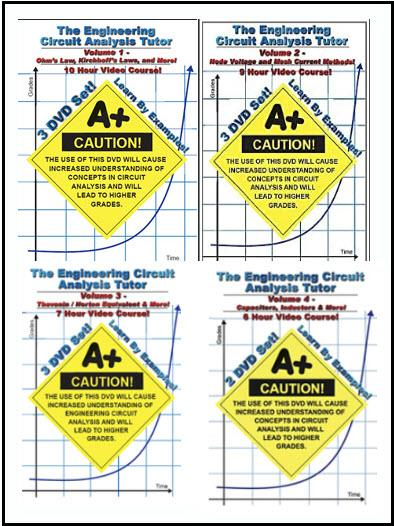Tutorials

# Math Tutor – Engineering Circuit Analysis: Vol.1,2,3,4

####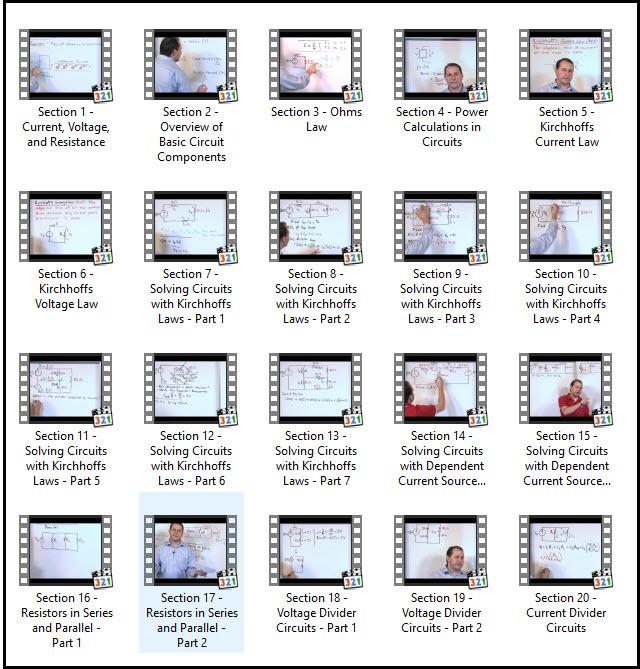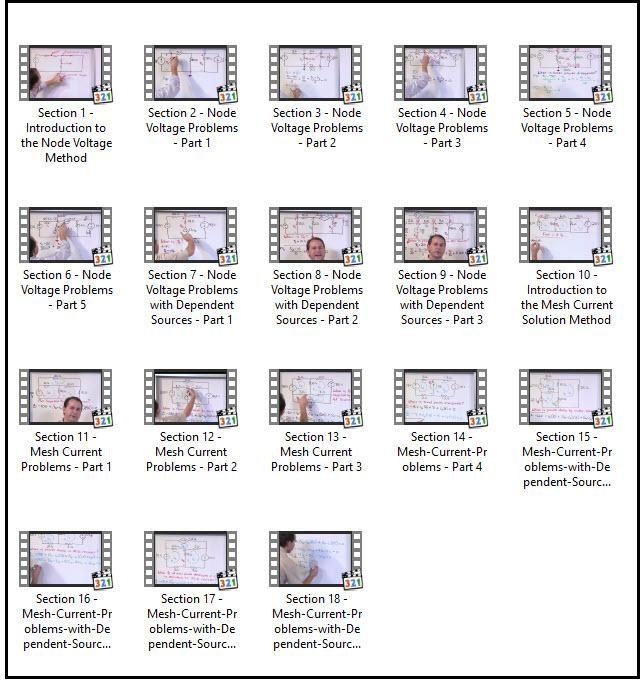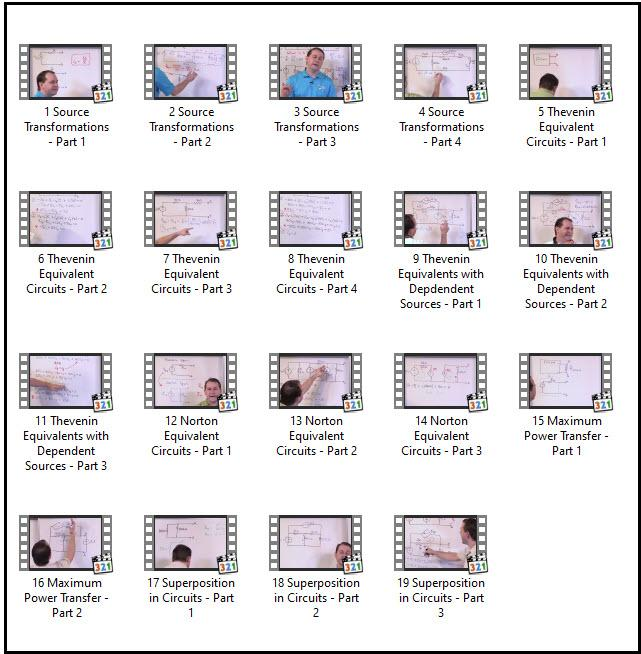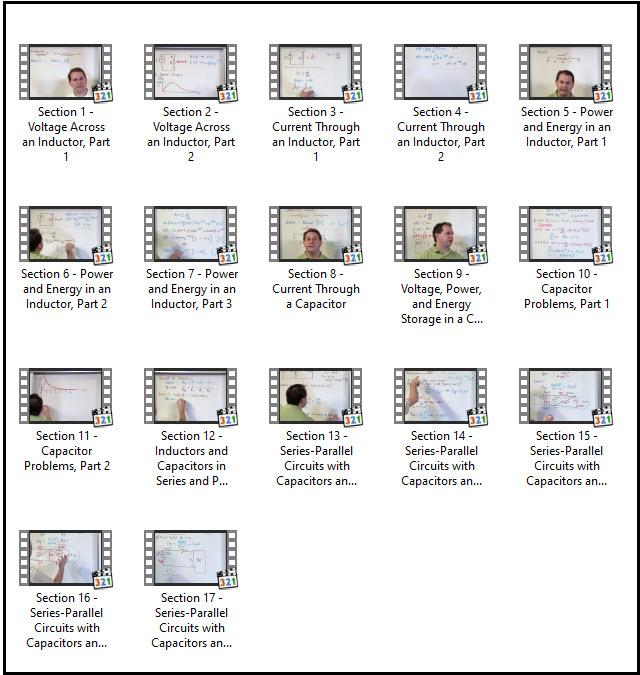#### Math Tutor – Engineering Circuit Analysis: Vol.1,2,3,4 –  5.42 GB

Engineering Circuit Analysis, also known as Circuit Theory, is difficult for most students primarily because the basic laws seem very simple. This leads the student to believe that the exam problems will not be too difficult, when in reality there is a large amount of technique in solving circuit problems. In other words, practicing circuit problems is absolutely essential to success in any circuits course.
We begin this course assuming that the student knows absolutely nothing about the solution methods to be learned. We introduce the concept of the inductor early in this course and show the relationship between current and voltage in an inductor. Next, we explore capacitors and show how they are constructed and why they are used in circuits.
Next, we solve circuits that involve capacitors and inductors and show how to simplify networks that have series and parallel arrangements. Every concept is taught by worked example problems that guides the student to mastery of these concepts.
With these tools, the student will be able to solve a wide variety of engineering level circuit problems with confidence.you must be registered member to see linkes Register Now

## You may also like...Tutorials

### Adam Thomas – Dropshipping Accelerator 2018Tutorials

### Gael Breton & Mark Webster Authority Hacker Pro (2020)Tutorials